# JP5773435B2 - Charger - Google Patents

## Info

Publication number
JP5773435B2
JP5773435B2 JP2011233821A JP2011233821A JP5773435B2 JP 5773435 B2 JP5773435 B2 JP 5773435B2 JP 2011233821 A JP2011233821 A JP 2011233821A JP 2011233821 A JP2011233821 A JP 2011233821A JP 5773435 B2 JP5773435 B2 JP 5773435B2
Authority
JP
Japan
Prior art keywords
correction
dc
power value
output
ripple
Prior art date
Legal status (The legal status is an assumption and is not a legal conclusion. Google has not performed a legal analysis and makes no representation as to the accuracy of the status listed.)
Active
Application number
JP2011233821A
Other languages
Japanese (ja)
Other versions
JP2013093952A (en
Inventor

Original Assignee
ニチコン株式会社
Priority date (The priority date is an assumption and is not a legal conclusion. Google has not performed a legal analysis and makes no representation as to the accuracy of the date listed.)
Filing date
Publication date
Application filed by ニチコン株式会社 filed Critical ニチコン株式会社
Priority to JP2011233821A priority Critical patent/JP5773435B2/en
Publication of JP2013093952A publication Critical patent/JP2013093952A/en
Application granted granted Critical
Publication of JP5773435B2 publication Critical patent/JP5773435B2/en
Application status is Active legal-status Critical
Anticipated expiration legal-status Critical

## Images

•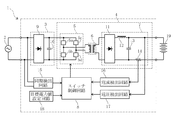•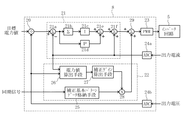•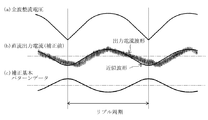•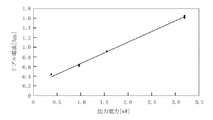•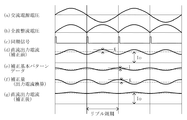•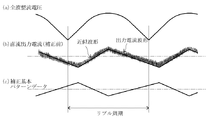## Description

The present invention relates to a charging device that converts AC power supplied from an AC power source into DC power and supplies the DC power to a battery.

Generally, a charging device includes a rectifying / smoothing circuit that rectifies and smoothes AC power supplied from an AC power source to generate DC input power, and DC / DC that switches DC input power to switch to DC output power by switching means. A converter circuit and a switch control circuit for controlling the duty ratio of the switch means are provided.

This charging device controls the duty ratio of the switch means according to the deviation between the power value of the DC output power output from the DC / DC converter circuit and the target power value, thereby providing a stable DC output power to the battery. To supply.

By the way, in the charging device provided with the switch means as described above, ripple may be included in the DC output power. If ripples are included in the DC output power, it will affect the battery life and, in turn, impose an excessive maintenance cost burden on the user.
For this reason, in a charging device, it is necessary to reduce the ripple in DC output power.

As a method for reducing the ripple in the DC output power, conventionally, a feed-forward that detects the DC input voltage input to the DC / DC converter circuit and controls the duty ratio of the switch means according to the ripple in the DC input voltage. A control method is known (see, for example, Patent Document 1).

In this conventional method, when the DC input voltage is increased, the duty ratio is decreased, while when the DC input voltage is decreased, the duty ratio is increased to reduce the ripple in the DC output power.

JP 2008-29089 A

However, since the conventional method employs the feedforward control method, when the DC output power increases or decreases due to load fluctuations, the increase or decrease is fed back and the ripple in the DC input power apparently increases or decreases.
That is, in the conventional method, when the load fluctuation occurs, the duty ratio of the switch means is controlled according to the ripple that is apparently increased or decreased, so that the DC output power output from the DC / DC converter circuit becomes unstable. There was a problem of becoming.

The present invention has been made in view of the above circumstances, and an object of the present invention is to provide a charging device capable of reducing ripple and supplying stable DC output power to a battery. .

In order to solve the above-described problems, a charging device according to the present invention includes a rectifying / smoothing circuit that rectifies and smoothes AC power supplied from an AC power source to generate DC input power, and switches DC input power with a switch unit. A DC / DC converter circuit for converting to DC output power and outputting to the battery, and a switch control circuit for feedback controlling the duty ratio of the switch means so that the output power value to the battery becomes a preset set power value; A charging device comprising:
It further includes a synchronization detection circuit that outputs a synchronization signal at the timing when the polarity of the AC power is inverted,
The switch control circuit
A control amount calculation unit for calculating an on / off control amount of the switch means by feedback control;
A correction amount calculation unit that calculates a correction amount based on a correction basic pattern that is synchronized with the synchronization signal, inverts the output ripple waveform output to the battery , and normalizes the amplitude magnitude to 1 ,
Have
By adding the correction amount calculated in the correction amount calculation unit on / off control amount calculated by the control amount calculating section have rows ripple correction processing for determining the duty ratio,
The correction amount calculation unit
Power value calculating means for calculating the output power value to the battery;
Correction for calculating a correction gain in the output power value calculated by the power value calculation means based on the correlation data indicating the correlation between the output power value to the battery and the ripple value when the ripple correction processing obtained in advance is not performed Gain calculation means;
Have
The correction amount is calculated by multiplying the correction basic pattern by the correction gain .

According to this configuration, the control amount calculation unit performs feedback control so that the output power value to the battery becomes the set power value, and the on / off control amount of the switch unit is calculated. The duty ratio is obtained by adding the correction amounts calculated by the correction amount calculation unit.
Here, a correction basic pattern is provided that includes a synchronization detection circuit that outputs a synchronization signal at the timing when the polarity of the AC power is inverted, and the correction amount calculation unit is synchronized with the synchronization signal and the output ripple waveform output to the battery is inverted. The correction amount is calculated based on the above.
That is, the correction amount is added to the on / off control amount so as to cancel the ripple included in the output to obtain the duty ratio, and the switch means is controlled by the duty ratio. For this reason, output ripple can be reduced and stable DC output power can be supplied to the battery.
Further, according to this configuration, the magnitude of the amplitude of the correction basic pattern is normalized to 1, and based on the correlation data indicating the correlation between the output power value and the ripple value when the ripple correction process is not performed. A correction amount can be calculated by calculating a correction gain in the output power value and multiplying the correction basic pattern by the correction gain. For this reason, an appropriate correction amount according to the output power value can be calculated, and output ripple can be reliably reduced.
Here, the interrelation data is data obtained in advance with respect to the interrelationship between the output power value and the ripple value when the duty ratio is controlled without adding the correction amount calculated by the correction amount calculation unit.

The correction amount calculation unit in the charging device is
Correction basic pattern data storage means for storing correction basic pattern data consisting of waveform data for at least one ripple period in DC output power;
The correction amount is calculated by multiplying the correction basic pattern stored in the correction basic pattern data storage means by the correction gain.

According to this configuration, the duty ratio is determined so as to surely cancel the output ripple by multiplying the correction basic pattern data including the waveform data corresponding to at least one ripple period in the DC output power by the correction gain. Can do.

Furthermore, it is preferable that the correction amount calculation unit calculates the correction amount by multiplying the correction basic pattern data by a correction gain in synchronization with the synchronization signal output from the synchronization detection circuit.

According to this configuration, since the ripple correction process is performed in synchronization with the AC power supply, low ripple output power can be stably supplied without causing a phase shift in the correction result.

Further, in the above charging device, the relationship between the output power value to the battery and the constant multiplied by the correction basic pattern that minimizes the ripple value in the output power value calculated by the power value calculation means is used as the correlation data. Find in advance,
The correction gain calculation means may read the constant in the output power value calculated by the power value calculation means from the correlation data to calculate the constant as the correction gain.

According to this configuration, the relationship between the output power value to the battery and the constant that is multiplied by the correction basic pattern that minimizes the ripple value in the output power value calculated by the power value calculation means is previously stored as the correlation data. Since the correction gain is calculated by reading the constant in the output power value calculated by the power value calculation means from the correlation data, the ripple can be minimized according to the output power value.

The charging device further includes a voltage detection circuit and a current detection circuit for detecting a DC output voltage and a DC output current output from the DC / DC converter circuit,
The power value calculation means calculates an output power value to the battery based on the voltage value and the current value detected by the voltage detection circuit and the current detection circuit.

According to this configuration, since the output power value to the battery is calculated based on the voltage value and the current value detected by the voltage detection circuit and the current detection circuit, an accurate output power value can be calculated.

The interrelation data may be a linear expression of the relationship between the output power value to the battery and the ripple value.

According to this configuration, since the relationship between the output power value to the battery and the ripple value is expressed by a linear expression, the ripple value according to the output power value can be easily calculated.

Further, the correction basic pattern may be a waveform obtained by approximating the output waveform of the DC / DC converter circuit by a cos function.

According to this configuration, the same function (cos function) can be used at any timing during one ripple period, and there is no need to switch the function according to the timing.

According to the present invention, it is possible to provide a charging device capable of reducing ripples and supplying stable DC output power to a battery.

It is a block diagram of the charging device which concerns on this invention. It is a block diagram of a switch control circuit in the present invention. It is a figure for demonstrating the correction | amendment basic pattern data in this invention. It is a figure which shows an example of the correlation data in this invention. It is a figure for demonstrating operation | movement of the charging device which concerns on this invention. It is a figure for demonstrating the modification of the correction | amendment basic pattern data in this invention.

Hereinafter, preferred embodiments of a charging device according to the present invention will be described with reference to the accompanying drawings.

[Configuration of charging device]
In FIG. 1, the block diagram of the charging device 1 which concerns on one Embodiment of this invention is shown.
As shown in the figure, a charging device 1 includes a rectifying / smoothing circuit 3 that rectifies and smoothes AC power supplied from an AC power source 2 such as a commercial AC power source to generate DC input power, and switches DC input power to switching means. DC / DC converter circuit 4 for switching to DC output power to be supplied to battery 19 by switching at 5a to 5d, and switch means 5a to 5 so that the output power value to battery 19 becomes a preset set power value. And a switch control circuit 8 for controlling the duty ratio of 5d.

The rectifying / smoothing circuit 3 includes a diode bridge circuit 9, an electrolytic capacitor 10 having a capacitance of several hundreds μF to several thousand μF, and a power factor correction circuit (not shown).
The DC / DC converter circuit 4 has an inverter circuit 5 composed of four switch means 5a to 5d such as IGBT and MOSFET, a booster circuit 6 composed of a transformer, and an output circuit 7 connected to the secondary side of the transformer. doing.
The output circuit 7 includes a diode bridge circuit 11, an LC low-pass filter including a coil 12 and a smoothing capacitor 13, and a shunt resistor 14 of several mΩ.

In addition, the charging device 1 according to the present embodiment includes a synchronization detection circuit 15 that outputs a synchronization signal at a timing when the polarity of the AC power (AC voltage) supplied from the AC power supply 2 is inverted, and a DC output that flows through the shunt resistor 14. A current detection circuit 16 that detects current, a voltage detection circuit 17 that detects a DC output voltage after passing through an LC low-pass filter, and a target power value setting circuit 18 that sets a target power value of DC output power are provided.

The synchronization signal output from the synchronization detection circuit 15, the current value detected by the current detection circuit 16, the voltage value detected by the voltage detection circuit 17, and the target power value set by the target power value setting circuit 18 are: Input to the control circuit 8.

The switch control circuit 8 is constituted by microcomputer software. As shown in FIG. 2, the switch control circuit 8 includes target current value calculation means 20 for calculating a target current value of the DC output current, and switch means 5a to 5d by feedback control. A control amount calculation unit 21 that calculates an on / off control amount, a correction amount calculation unit 22 that calculates a correction amount corresponding to an on / off control amount corresponding to a ripple value in DC output power, and a correction to an on / off control amount An arithmetic means 29 for adding the amount, a PWM signal generating means 23 for generating a PWM signal for controlling the duty ratio of the switch means 5a to 5d, and AD converting means 24a, 24b for converting the analog signal into a digital signal. Have.

The target current value calculation means 20 calculates a target current value based on the target power value set by the target power value setting circuit 18 and the voltage value of the DC output voltage detected by the voltage detection circuit 17.
Usually, since the battery 19 has an extremely low resistance, the DC output voltage is almost equal to the battery voltage. The DC output voltage is constant at least during the feedback control period.
Therefore, the expression “target power value / voltage value of DC output voltage = target current value” is established.

The control amount calculator 21 includes a deviation calculator 21a that calculates a deviation between the target current value calculated by the target current value calculator 20 and the current value digitized by the AD converter 24a, and the calculated deviation. Integral calculating means 21b for integrating the integrated deviation, integral gain calculating means 21c for multiplying the integrated deviation by a proportional constant obtained in advance through experiments or simulations, proportional gain calculating means 21d for multiplying the deviation by another proportional constant, A first control amount calculation means 21e for adding the calculation result of the proportional gain calculation means 21d to the calculation result of the integral gain calculation means 21c, and adding the current value to the calculation result of the first control amount calculation means 21e to turn on / off And a second control amount calculating means 21f for calculating an off control amount.

The correction amount calculation unit 22 includes a correction basic pattern data storage unit 25, a power value calculation unit 26, a correction gain calculation unit 27, and a correction amount calculation unit 28.

The correction basic pattern data storage means 25 is a database in which correction basic pattern data for one or more ripple periods in DC output power is stored in advance.
As shown in FIG. 3, the ripple period in the corrected basic pattern data (see FIG. 3C) is the same as the period of the voltage waveform (see FIG. 3A) that has been full-wave rectified by the diode bridge circuit 9. .
The corrected basic pattern data is obtained by normalizing the amplitude of the output ripple waveform (approximate waveform of the DC output current waveform (see FIG. 3B)) by inverting the polarity up and down. Therefore, if the approximate waveform of the DC output current waveform is a cos function waveform, the correction basic pattern data also has a cos function waveform.

The power value calculation means 26 calculates the current power value of the DC output power supplied to the battery 19 based on the current value and the voltage value detected by the current detection circuit 16 and the voltage detection circuit 17.

The correction gain calculation means 27 shows a linear relationship between the power value of the DC output power when the ripple correction processing previously obtained by the power value calculation means 26 is not performed and the ripple current value in the DC output power as a linear expression. A ripple current value for the current power value is predicted based on the correlation data (see FIG. 4), and a correction gain is calculated based on the predicted ripple current value.
Note that the correction gain calculation means 27 stores a plurality of pieces of correlation data that differ depending on conditions such as temperature.

The correction amount calculation unit 28 adds the correction gain calculated by the correction gain calculation unit 27 to the correction basic pattern data stored in the correction basic pattern data storage unit 25 every time a synchronization signal is output from the synchronization detection circuit 15. The correction amount is calculated by multiplication, and the correction amount is output to the calculation means 29.

The calculating unit 29 adds the correction amount calculated by the correction amount calculating unit 28 to the on / off control amount calculated by the control amount calculating unit 21.

The PWM signal generation unit 23 generates a PWM signal for controlling the duty ratio of the switch units 5a to 5d based on the on / off control amount to which the correction amount is added.

[Operation of charging device]
Next, the operation of the charging device 1 according to this embodiment will be specifically described.
In this specific example, a case where a ripple of magnitude K is included in the DC output current output from the output circuit 7 without performing the ripple correction process (see FIG. 5D) will be described.

In the charging device 1, the AC power supply voltage (see FIG. 5A) supplied from the AC power supply 2 is full-wave rectified by the diode bridge circuit 9 (see FIG. 5B) and smoothed by the electrolytic capacitor 10. Thus, it is converted into a DC input voltage and supplied to the DC / DC converter circuit 4.

The DC input voltage supplied to the DC / DC converter circuit 4 is converted into a primary AC voltage higher than the frequency of the AC power supply 2 (several tens of kHz) by the switch means 5a to 5d of the inverter circuit 5, and then boosted. The voltage is boosted by the circuit 6 and supplied to the output circuit 7 as a secondary AC voltage.

The secondary side AC voltage supplied to the output circuit 7 is converted again to a DC voltage by the diode bridge circuit 11, and then the switching noise of several tens of kHz generated in the switch means 5a to 5d is removed by the LC low-pass filter. The output voltage is supplied to the battery 19.

Here, the magnitude of the ripple in the DC output current is calculated (predicted) by the correction gain calculating unit 27 based on the current power value calculated by the power value calculating unit 26 and the correlation data shown in FIG. Is done. For example, FIG. 4 shows that when the current power value is 1.5 kW, the ripple value is 0.9 (App).

Based on the ripple value calculated by the correction gain calculation means 27, the correction gain for making the amplitude of the correction basic pattern data (see FIG. 5E) stored in the correction basic pattern data storage means 25 K times. K is calculated.
In general, the correction gain has a proportional relationship with the ripple value, but here, for the sake of simplicity, the correction gain will be described as the ripple value.

When the correction gain K is calculated by the correction gain calculator 27, the correction amount calculator 28 converts the correction basic pattern data into the correction basic pattern data in synchronization with the synchronization signal (see FIG. 5C) output from the synchronization detection circuit 15. A correction amount (see FIG. 5 (f)) multiplied by the correction gain K is calculated every moment, and the correction amount is output to the calculation means 29.

Since the correction basic pattern data stored in the correction basic pattern data storage means 25 is a normalized waveform of the cos function having a size of 1, the correction amount is K (see FIG. 5F). ).
Therefore, for example, when the current power value is 1.5 kW, the magnitude of the correction amount is 0.9.

In the calculating means 29, the correction amount calculated by the correction amount calculating means 28 is added to the on / off control amount calculated by the control amount calculating section 21, and the calculation result is output to the PWM signal generating means 23. .

The PWM signal generation unit 23 generates a PWM signal based on the calculation result (on / off control amount with the correction amount added).
Thereby, the duty ratio of the switch means 5a-5d is changed, and the ripple of DC output current is reduced (refer FIG.5 (g)).

Eventually, according to the charging device 1 according to the present embodiment, the duty ratios of the switch units 5a to 5d are controlled based on the on / off control amounts of the switch units 5a to 5d to which the correction amount corresponding to the ripple value is added. Therefore, ripples can be reduced and stable DC output power can be supplied to the battery 19.

Moreover, in the charging device 1 according to the present embodiment, the capacitance of the electrolytic capacitor 10 can be reduced without increasing the ripple value. For this reason, according to the charging device 1 which concerns on this embodiment, size reduction and cost reduction of the whole apparatus are realizable.

As mentioned above, although preferable embodiment of the charging device which concerns on this invention was described, this invention is not limited to the said embodiment.

For example, in the above embodiment, the approximate waveform of the DC output current waveform is the waveform of the cos function, and the correction basic pattern data is also the waveform of the cos function. However, as shown in FIG. The waveform of a function can be used, and the correction basic pattern data can also be a waveform of a broken line function.
By making the approximate waveform of the DC output current waveform and the corrected basic pattern data into a waveform of a polygonal line function, ripples can be almost completely eliminated.
When the correction basic pattern data is a polygonal line function waveform, there are a plurality of functions (calculation formulas) in one ripple period, and therefore, it is necessary to switch the function for each inflection point. On the other hand, when the correction basic pattern data is a waveform of the cos function, there is only one function in one ripple period, so the same function can be used at any timing and calculation is easy. There is an advantage of becoming.

Further, instead of storing the correction basic pattern data of the cos function or the polygonal line function in the correction basic pattern data storage means 25, a table in which the calculation results of the cos function and the polygonal line function calculated for each feedback control period are tabulated is corrected. The pattern data may be stored in the corrected basic pattern data storage means 25.

Furthermore, in the above embodiment, the correction basic pattern data is obtained by inverting the polarity of the approximate waveform of the DC output current waveform, so the calculation means 29 adds the correction amount to the on / off control amount. When the basic correction pattern data is not the above-mentioned polarity inverted (when the polarities are the same), the calculation means 29 needs to subtract the correction amount from the on / off control amount.

In the above embodiment, the correction gain K is set to the ripple value, and the correction gain K is multiplied by the correction basic pattern data to calculate the correction amount. However, when the correction gain K is set to the ripple value, the ripple is completely eliminated. If this is not possible, a constant K ′ (= ripple value × proportional constant) that minimizes the ripple current value at the current output power value calculated by the power value calculating means 26 may be used as the correction gain. .
The proportionality constant multiplied by the ripple value can be calculated based on, for example, the difference between the correction amount calculated as the correction gain K = ripple value and the ripple current value at the current output power value.
When the constant K ′ (= ripple value × proportional constant) is used as the correction gain, the linear relationship between the power value of the DC output power obtained in advance by the power value calculation means 26 and the constant K ′ in the DC output power is a linear expression. Is stored in the correction gain calculating means 27, and a constant K ′ corresponding to the current output power value is calculated as a correction gain from the correlation data.

Note that the present invention can be applied not only to a vehicle-mounted charging device mounted on an electric vehicle, but also to charging devices in other fields.

DESCRIPTION OF SYMBOLS 1 Charging device 2 AC power supply 3 Rectification smoothing circuit 4 DC / DC converter circuit 5 Inverter circuits 5a to 5d Switch means 6 Booster circuit 7 Output circuit 8 Switch control circuit 9 Diode bridge circuit 10 Electrolytic capacitor 11 Diode bridge circuit 12 Coil 13 Smoothing capacitor 14 shunt resistor 15 synchronization detection circuit 16 current detection circuit 17 voltage detection circuit 18 target power value setting circuit 19 battery 20 target current value calculation means 21 control amount calculation section 22 correction amount calculation section 23 PWM signal generation means 24a, 24b AD conversion means 25 correction basic pattern data storage means 26 power value calculation means 27 correction gain calculation means 28 correction amount calculation means 29 calculation means

## Claims (7)

1. A rectifying / smoothing circuit that rectifies and smoothes AC power supplied from an AC power source to generate DC input power, and DC / DC that switches the DC input power by a switching means to convert it to DC output power and outputs the DC power to a battery. A charging device comprising: a converter circuit; and a switch control circuit that feedback-controls the duty ratio of the switch means so that an output power value to the battery becomes a preset set power value,
A synchronization detection circuit that outputs a synchronization signal at a timing at which the polarity of the AC power is inverted;
The switch control circuit includes:
A control amount calculation unit for calculating an on / off control amount of the switch means by the feedback control;
A correction amount calculation unit that calculates a correction amount based on a correction basic pattern that is synchronized with the synchronization signal, inverts an output ripple waveform that is output to the battery , and that is normalized to a magnitude of 1 ;
Have
There line ripple correction processing for obtaining the duty ratio by adding the correction amount calculated in the correction amount calculation unit on / off control amount calculated by the control amount calculating section,
The correction amount calculation unit
Power value calculating means for calculating an output power value to the battery;
The correction gain in the output power value calculated by the power value calculation means based on the correlation data indicating the correlation between the output power value to the battery and the ripple value when the ripple correction processing that has been obtained in advance is not performed. Correction gain calculating means for calculating;
Have
The charging device , wherein the correction amount is calculated by multiplying the correction basic pattern by the correction gain .
2. The correction amount calculation unit
Correction basic pattern data storage means for storing the correction basic pattern data consisting of waveform data of at least one ripple period in the DC output power;
The charging device according to claim 1, wherein the correction amount is calculated by multiplying the correction basic pattern stored in the correction basic pattern data storage unit by the correction gain.
3. The correction amount calculation unit calculates the correction amount by multiplying the correction basic pattern data by the correction gain in synchronization with the synchronization signal output from the synchronization detection circuit. The charging device according to 1 or 2.
4. A relationship between the output power value to the battery and a constant that multiplies the correction basic pattern that minimizes the ripple value in the output power value calculated by the power value calculation unit is obtained in advance as the interrelated data. Every
The correction gain calculation means reads the constant in the output power value calculated by the power value calculation means from the correlation data, thereby calculating the constant as the correction gain. 4. The charging device according to any one of 3.
5. A voltage detection circuit and a current detection circuit for detecting a DC output voltage and a DC output current output from the DC / DC converter circuit, respectively;
5. The power value calculating means calculates an output power value to the battery based on a voltage value and a current value detected by the voltage detection circuit and the current detection circuit. A charging device according to claim 1.
6. 6. The charging device according to claim 1, wherein the correlation data is a linear expression of a relationship between an output power value to the battery and a ripple value.
7. The charging device according to claim 1, wherein the correction basic pattern is a waveform obtained by approximating an output waveform of the DC / DC converter circuit by a cos function.
JP2011233821A 2011-10-25 2011-10-25 Charger Active JP5773435B2 (en)

## Priority Applications (1)

Application Number Priority Date Filing Date Title
JP2011233821A JP5773435B2 (en) 2011-10-25 2011-10-25 Charger

## Applications Claiming Priority (1)

Application Number Priority Date Filing Date Title
JP2011233821A JP5773435B2 (en) 2011-10-25 2011-10-25 Charger

## Publications (2)

Publication Number Publication Date
JP2013093952A JP2013093952A (en) 2013-05-16
JP5773435B2 true JP5773435B2 (en) 2015-09-02

# Family

## Family Applications (1)

Application Number Title Priority Date Filing Date
JP2011233821A Active JP5773435B2 (en) 2011-10-25 2011-10-25 Charger

## Country Status (1)

JP (1) JP5773435B2 (en)

## Families Citing this family (2)

* Cited by examiner, † Cited by third party
Publication number Priority date Publication date Assignee Title
US9882466B2 (en) 2014-11-11 2018-01-30 Mitsubishi Electric Corporation Power conversion device including an AC/DC converter and a DC/DC converter
JP6589046B2 (en) * 2016-02-05 2019-10-09 グァンドン オッポ モバイル テレコミュニケーションズ コーポレーション リミテッドＧｕａｎｇｄｏｎｇ Ｏｐｐｏ Ｍｏｂｉｌｅ Ｔｅｌｅｃｏｍｍｕｎｉｃａｔｉｏｎｓ Ｃｏｒｐ．， Ｌｔｄ． Adapter and charge control method

## Family Cites Families (3)

* Cited by examiner, † Cited by third party
Publication number Priority date Publication date Assignee Title
JPH05292741A (en) * 1992-04-09 1993-11-05 Kageki Matsui Forward converter for improving higher harmonic characteristics of power supply
AT173570T (en) * 1995-07-31 1998-12-15 Hewlett Packard Co Clocked switching power supply with power factor correction
JP2732428B2 (en) * 1995-09-08 1998-03-30 西芝電機株式会社 Chopper device

## Also Published As

Publication number Publication date
JP2013093952A (en) 2013-05-16

## Similar Documents

Publication Publication Date Title
US8653413B2 (en) Welding power supply with digital control of duty cycle
US8630105B2 (en) Switching power supply apparatus including a PFC converter that suppresses harmonic currents
EP2437909B1 (en) Welding power supply for and method of determining during welding a weld cable inductance
US7852058B2 (en) Power supply regulator with digital control
JP5182375B2 (en) PFC converter
JP3969390B2 (en) Switching power supply
Mattavelli et al. Analysis of control-delay reduction for the improvement of UPS voltage-loop bandwidth
EP2919374B1 (en) Duty-ratio controller
GB2253311A (en) Power factor correction converter
DE102012208880A1 (en) AC / DC converter with a PFC and a DC / DC converter
US8094473B2 (en) Bridgeless power factor correction circuit
JP2010114993A (en) Control system of power factor correction circuit
US8525495B2 (en) Input current generator for buck-boost circuit control
JPWO2010061652A1 (en) PFC converter
JP2005110434A (en) Power factor improvement circuit
CN102656787B (en) Switching power supply circuit and power factor controller
JP2006094696A (en) Power factor correcting circuit and its output voltage control method
CN103956905A (en) System and method for adjusting output currents in power source transformation system
US20040252532A1 (en) Power supply apparatus
JP4972142B2 (en) Power factor correction apparatus and control method thereof
KR100963309B1 (en) Power supply system and method for controlling output voltage
JP2006025531A (en) Dc/dc converter circuit
EP2681833B1 (en) Controlling a switched mode power supply with maximised power efficiency
CN103477294B (en) Power conditioner system
WO2014199796A1 (en) Inverter device

## Legal Events

Date Code Title Description
A621 Written request for application examination

Free format text: JAPANESE INTERMEDIATE CODE: A621

Effective date: 20140409

A977 Report on retrieval

Free format text: JAPANESE INTERMEDIATE CODE: A971007

Effective date: 20150204

A131 Notification of reasons for refusal

Free format text: JAPANESE INTERMEDIATE CODE: A131

Effective date: 20150210

A521 Written amendment

Free format text: JAPANESE INTERMEDIATE CODE: A523

Effective date: 20150312

TRDD Decision of grant or rejection written
A01 Written decision to grant a patent or to grant a registration (utility model)

Free format text: JAPANESE INTERMEDIATE CODE: A01

Effective date: 20150624

A61 First payment of annual fees (during grant procedure)

Free format text: JAPANESE INTERMEDIATE CODE: A61

Effective date: 20150625

R150 Certificate of patent or registration of utility model

Ref document number: 5773435

Country of ref document: JP

Free format text: JAPANESE INTERMEDIATE CODE: R150

R250 Receipt of annual fees

Free format text: JAPANESE INTERMEDIATE CODE: R250

R250 Receipt of annual fees

Free format text: JAPANESE INTERMEDIATE CODE: R250